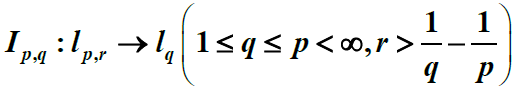# 无穷维恒等算子的Kolmogorov n-宽度Kolmogorov n-Width of Infinite Dimension Identity Operator

• 全文下载: PDF(476KB)    PP.519-524   DOI: 10.12677/AAM.2018.75063
• 下载量: 571  浏览量: 853   国家自然科学基金支持

In this paper, we study the Kolmogorov n-width of infinite dimension identity operator, and obtain its asymptotic degree.

1. 引言及主要结果

${d}_{n}\left(W,Z\right)=\underset{{F}_{n}}{\mathrm{inf}}\underset{x\in W}{\mathrm{sup}}\underset{y\in {F}_{n}}{\mathrm{inf}}‖x-y‖$

${d}_{n}\left(T:X\to Y\right)={d}_{n}\left(T\left({B}_{z}\right),\stackrel{¯}{Y}\right)$

$1\le p\le \infty$ ，对任一实序列 $x={\left\{{x}_{n}\right\}}_{n=1}^{\infty }$ ，令

${‖x‖}_{{l}_{p}}=\left\{\begin{array}{l}{\left(\underset{n=1}{\overset{\infty }{\sum }}{|{x}_{n}|}^{p}\right)}^{1/p},\text{ }1\le p<\infty \\ \underset{n\ge 1}{\mathrm{sup}}|{x}_{n}|,\text{ }\text{ }\text{\hspace{0.17em}}\text{\hspace{0.17em}}\text{\hspace{0.17em}}\text{\hspace{0.17em}}\text{\hspace{0.17em}}p=\infty \end{array}$

${l}_{p}$ 表示满足条件 ${‖x‖}_{{l}_{p}}<\infty$ 的实序列x所构成的集合。众所周知， ${‖·‖}_{{l}_{p}}$${l}_{p}$ 上的一个范数。而且 ${l}_{p}$ 是一个Banach空间。易见， ${l}_{p}$ 空间具有如下性质：

1) ${l}_{p}\subset {l}_{q}$ $\left(1\le p\le q\le \infty \right)$

2) ${l}_{p}\not\subset {l}_{q}$ $\left(1\le q

${x}^{r}:={\left\{{n}^{r}{x}_{n}\right\}}_{n=1}^{\infty }$${‖x‖}_{{l}_{p,r}}={‖{x}^{\left(r\right)}‖}_{{l}_{p}}$

${l}_{p,r}:=\left\{x\in {l}_{p}|{‖x‖}_{{l}_{p,r}}<\infty \right\}$

$1\le q$r>\frac{1}{q}-\frac{1}{p}$ ，( $p=\infty$ 时，记 $\frac{1}{p}=0$ )。对任意的 $x=\left\{{x}_{n}\right\}\in {l}_{p,r}$ ，由Hölder不等式有

${‖x‖}_{{l}_{q}}\le \left\{\begin{array}{l}{‖x‖}_{{l}_{p.r}}{\left(\underset{n=1}{\overset{\infty }{\sum }}{n}^{-\frac{pr}{p-q}}\right)}^{\frac{1}{q}-\frac{1}{p}}<\infty ,\text{ }1\le q

${I}_{p,q}:{l}_{p,r}\to {l}_{q}$

$x↦x$

${l}_{p,r}$${l}_{q}$ 的有界线性算子。

${d}_{n}\left({I}_{p,q}:{l}_{p,r}\to {l}_{q}\right)\succ \prec {n}^{-\left(r-\frac{1}{q}+\frac{1}{p}\right)}$

2. 主要结果的证明

${ℝ}^{m}=\left\{x=\left({x}_{1},\cdots ,{x}_{m}\right)|{x}_{i}\in R,i=1,\cdots ,m\right\}$

$1\le p\le \infty$$x=\left({x}_{1},\cdots ,{x}_{m}\right)\in {ℝ}^{m}$ 。令

${‖x‖}_{{l}_{p}^{m}}=\left\{\begin{array}{l}{\left(\underset{n=1}{\overset{m}{\sum }}{|{x}_{n}|}^{p}\right)}^{1/p},\text{ }1\le p<\infty ,\\ \underset{1\le n\le m}{\mathrm{max}}|{x}_{n}|,\text{ }\text{ }\text{ }p=\infty \end{array}$

${‖·‖}_{{l}_{p}^{m}}$${ℝ}^{m}$ 上的范数。用 ${l}_{p}^{m}$ 表示 ${ℝ}^{m}$ 按照范数 ${‖·‖}_{{l}_{p}^{m}}$ 所构成的Banach空间。用 ${B}_{p}^{m}$ 表示 ${l}_{p}^{m}$ 中的单位球。易见 ${\left\{{{e}^{\prime }}_{n}\right\}}_{n=1}^{m}$${l}_{p}^{m}$ 的基，其中 ${e}_{n}^{}{}^{\prime }=\left(0,\cdots ,0,1,0,\cdots ,0\right)\in {ℝ}^{m}$ (第n个分量为1，其余分量为0)。

${d}_{n}\left({B}_{p}^{m},{l}_{q}^{m}\right)=\left\{\begin{array}{l}{\left(m-n\right)}^{\frac{1}{q}-\frac{1}{p}},\text{\hspace{0.17em}}\text{\hspace{0.17em}}0\le n\le m\\ 0,\text{\hspace{0.17em}}\text{\hspace{0.17em}}\text{\hspace{0.17em}}\text{\hspace{0.17em}}\text{\hspace{0.17em}}\text{\hspace{0.17em}}\text{\hspace{0.17em}}\text{\hspace{0.17em}}\text{\hspace{0.17em}}\text{\hspace{0.17em}}\text{\hspace{0.17em}}\text{\hspace{0.17em}}\text{\hspace{0.17em}}\text{\hspace{0.17em}}\text{\hspace{0.17em}}\text{\hspace{0.17em}}\text{\hspace{0.17em}}n>m\end{array}$

$\forall k\in ℕ$ ，其中 $ℕ=\left\{1,2,\cdots \right\}$ ，记 ${S}_{k}=\left\{n\in N|{2}^{k-1}\le n<{2}^{k}\right\}$ 。则对任意的 $k,{k}^{\prime }\in ℕ$ ，且 ${S}_{k}\cap {S}_{{k}^{\prime }}=\varnothing$$ℕ=\underset{k=1}{\overset{\infty }{\cup }}{S}_{k}$ 。用 ${m}_{k}$ 表示 ${S}_{k}$ 中元素的个数，则 ${m}_{k}=|{S}_{k}|={2}^{k-1}$

$k\in ℕ$ ，记 ${F}_{k}=span\left\{{e}_{n}|n\in {S}_{k}\right\}$ ，则 $\mathrm{dim}{F}_{k}={m}_{k}={2}^{k-1}$ 。令

${I}_{k}:{F}_{k}\to {R}^{{m}_{k}}$

$x=\underset{n\in {S}_{k}}{\sum }{x}_{n}{e}_{n}↦\underset{j=1}{\overset{m}{\sum }}{x}_{{2}^{k-1}+j-1}{{e}^{\prime }}_{{2}^{k-1}+j-1}$

$\begin{array}{c}{‖x‖}_{{l}_{p,r}}={\left(\underset{n\in {S}_{k}}{\sum }{|{n}^{r}{x}_{n}|}^{p}\right)}^{1/p}\succ \prec {\left(\underset{n\in {S}_{k}}{\sum }{|{2}^{rk}{x}_{n}|}^{p}\right)}^{1/p}\\ ={2}^{rk}{\left(\underset{n\in {S}_{k}}{\sum }{|{x}_{n}|}^{p}\right)}^{1/p}={2}^{rk}{‖{I}_{k}x‖}_{{l}_{p}^{{m}_{k}}}\end{array}$ (2.1)

${‖x‖}_{{l}_{p}}={\left(\underset{n\in {S}_{k}}{\sum }{|{x}_{n}|}^{p}\right)}^{1/p}={‖{I}_{k}x‖}_{{l}_{p}^{{m}_{k}}}.$ (2.2)

${d}_{n}\left({B}_{p,r},{l}_{q}\right)\ll \underset{n=1}{\overset{\infty }{\sum }}{2}^{-rk}{d}_{{n}_{k}}\left({B}_{p}^{{m}_{k}},{l}_{q}^{{m}_{k}}\right).$

$1\ge {‖x‖}_{{l}_{p,r}}\gg {2}^{rk}{‖{I}_{k}x‖}_{{l}_{p}^{{m}_{k}}}$

$\forall y\in {F}_{k}$ ，由(2.2)知

${‖y‖}_{{l}_{q}}={‖{I}_{k}y‖}_{{l}_{q}^{{m}_{k}}}$

${d}_{{n}_{k}}\left({B}_{p,r}\cap {F}_{k},{l}_{q}\cap {F}_{k}\right)\ll {2}^{-rk}{d}_{{n}_{k}}\left({B}_{p}^{{m}_{k}},{l}_{q}^{{m}_{k}}\right)$

$\underset{x\in {B}_{p,r}\cap {F}_{k}}{\mathrm{sup}}\underset{y\in {M}_{k}}{\mathrm{inf}}{‖x-y‖}_{{l}_{q}}\ll {2}^{-rk}{d}_{{n}_{k}}\left({B}_{p}^{{m}_{k}},{l}_{q}^{{m}_{k}}\right)$

$M=\underset{k=1}{\overset{\infty }{\sum }}{M}_{k}$ (直和)。则M为 ${l}_{q}$ 的线性子空间，且

$\mathrm{dim}M\le \underset{k=1}{\overset{\infty }{\sum }}\mathrm{dim}{M}_{k}\le \underset{k=1}{\overset{\infty }{\sum }}{n}_{k}\le n.$

$\begin{array}{c}{d}_{n}\left({B}_{p,r},{l}_{q}\right)\ll \underset{x\in {B}_{p,r}}{\mathrm{sup}}\underset{y\in M}{\mathrm{inf}}{‖x-y‖}_{{l}_{q}}\\ \le \underset{k=1}{\overset{\infty }{\sum }}\underset{x\in {B}_{p,r}\cap {F}_{k}}{\mathrm{sup}}\underset{y\in {M}_{k}}{\mathrm{inf}}{‖x-y‖}_{{l}_{q}}\\ \ll \underset{k=1}{\overset{\infty }{\sum }}{2}^{-rk}{d}_{{n}_{k}}\left({B}_{p}^{{m}_{k}},{l}_{q}^{{m}_{k}}\right)\end{array}$

${d}_{n}\left({B}_{p,r},{l}_{q}\right)\gg {2}^{-rk}{d}_{n}\left({B}_{p}^{{m}_{k}},{l}_{q}^{{m}_{k}}\right)$

$1\ge {‖x‖}_{{l}_{p}^{{m}_{k}}}\ge {2}^{rk}{‖{I}_{k}{}^{-1}x‖}_{{l}_{p,r}}$

$\forall y\in {l}_{q}^{{m}_{k}}$ ，则由(2.2)有

${‖y‖}_{{l}_{q}^{{m}_{k}}}={‖{I}_{k}{}^{-1}y‖}_{{l}_{q}}$

${d}_{n}\left({B}_{p,r},{l}_{q}\right)\ge {d}_{n}\left({B}_{p,r}\cap {F}_{k},{l}_{q}\cap {F}_{k}\right)\gg {2}^{-rk}{d}_{n}\left({B}_{p}^{{m}_{k}},{l}_{q}^{{m}_{k}}\right)$

$\forall k\in ℕ$ ，令 ${k}^{\prime }=\left[\mathrm{lg}n\right]$

${n}_{k}=\left\{\begin{array}{l}{m}_{k},\text{ }\text{\hspace{0.17em}}\text{\hspace{0.17em}}\text{\hspace{0.17em}}\text{\hspace{0.17em}}\text{\hspace{0.17em}}\text{\hspace{0.17em}}\text{\hspace{0.17em}}\text{\hspace{0.17em}}\text{\hspace{0.17em}}1\le k<{k}^{\prime }\\ {2}^{{k}^{\prime }}\cdot {2}^{\beta \left({k}^{\prime }-k\right)},\text{ }k\ge {k}^{\prime }\end{array}$

$\begin{array}{l}{d}_{n}\left({I}_{p,q}:{l}_{p,r}\to {l}_{q}\right)\\ \ll \underset{n=1}{\overset{\infty }{\sum }}{2}^{-rk}{d}_{{n}_{k}}\left({B}_{p}^{{m}_{k}},{l}_{q}^{{m}_{k}}\right)=\underset{k={k}^{\prime }}{\overset{\infty }{\sum }}{2}^{-rk}{d}_{{n}_{k}}\left({B}_{p}^{{m}_{k}},{l}_{q}^{{m}_{k}}\right)\\ \ll \underset{k={k}^{\prime }}{\overset{\infty }{\sum }}{2}^{-rk}\cdot {2}^{\left(\frac{1}{q}-\frac{1}{p}\right)k}=\underset{k={k}^{\prime }}{\overset{\infty }{\sum }}{2}^{-\left(r-\frac{1}{q}+\frac{1}{p}\right)k}\ll {2}^{-\left(r-\frac{1}{q}+\frac{1}{p}\right){k}^{\prime }}\ll {n}^{-\left(r-\frac{1}{q}+\frac{1}{p}\right)}\end{array}$

${d}_{n}\left({I}_{p,q}:{l}_{p,r}\to {l}_{q}\right)\gg {2}^{-rk}{d}_{n}\left({B}_{p}^{{m}_{k}},{l}_{q}^{{m}_{k}}\right)\gg {2}^{-rk}\cdot {n}^{\frac{1}{q}-\frac{1}{p}}\gg {n}^{-\left(r-\frac{1}{q}+\frac{1}{p}\right)}$

  Traub, J.F. Wasilkowski, G.W. and Wozniakowski, H. (1988) Information-Based Complexity. Academic Press, Boston, 1988.  Kolmogorov, A.N. (1936) Uber die deste Annaherung yon funktionen einer gegebenen funktioneklasse. Annals of Mathematics, 37, 107-111. 〈br/〉https://doi.org/10.2307/1968691  Stechkin, S.R. (1954) On Best Approximation of Given Classes of Functions by Arbitrary Polynomials. Uspekhi Matematicheskikh Nauk, 9, 133-134. (In Russian)  Tikhomirov, V.M. (1960) Diameters of Sets in Function Spaces and the Theory of Best Approximations. Uspekhi Matematicheskikh Nauk, 15, 81-120.  Pietsch, A. (1974) s-Numbers of Operators in Banach Spaces. Studia Mathematica, 51, 201-223. 〈br/〉https://doi.org/10.4064/sm-51-3-201-223  Stesin, M.I. (1975) Aleksandrov Widths of Finite—Dimensional Sets and Classes of Smooth Functions. Doklady Akademii Nauk, 220, 1278-1281.  Ismagilov, R.S. (1974) Widths of Sets in Normed Linear Spaces and Approximation of Functions by Trigonometric Polynomials. Uspekhi Matematicheskikh Nauk, 29, 161-178.  Pinkus, A. (1985) n-Widths in Approximation Theory. Springer, Berlin.